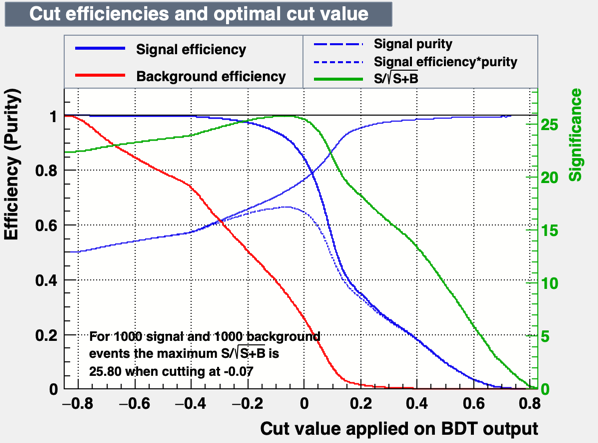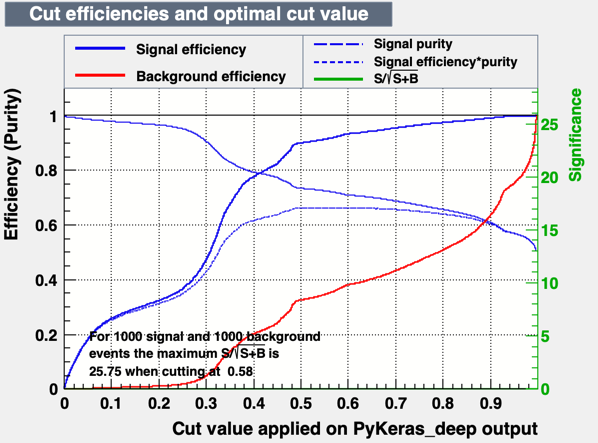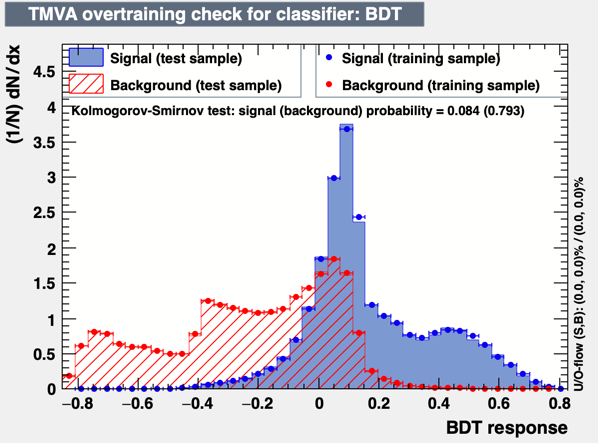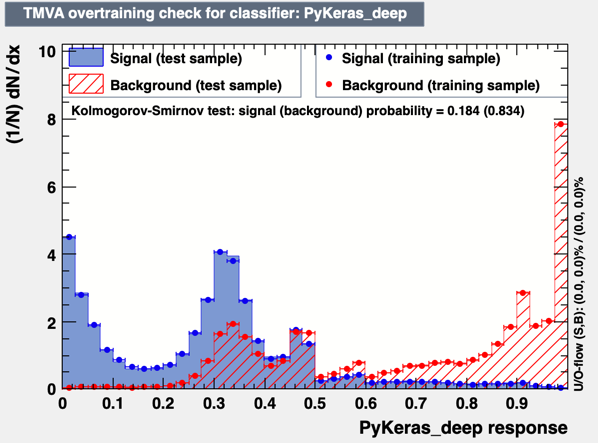# PyKeras training has a strange behaviour compared to BDT

Dear TMVA experts,
I’m using TMVA for a signal/background classification problem. In particular, I’m using several trees with signal samples and a single tree with background samples (drell-yan).
In the classification macro, I try different methods for training:

• PyKeras (with a python script that defines the model architecture)
• BDTs

Using the BDT approach, I see something like that:The code for the BDT option is:

``````factory.BookMethod(dataloader, TMVA.Types.kBDT, "BDT",
``````

When I use the PyKeras code, instead, I see something like:From this plot, I don’t understand why the green line is stuck at 0 (not showing) and why the blue and red line start at zero. In the BDT plot, instead, with no cut, the efficiency is 1 which makes more sense to me.

The code where the network is defined is:

``````        factory.BookMethod(dataloader, TMVA.Types.kPyKeras, 'PyKeras_deep', 'VarTransform=N:FilenameModel=model_deep.h5:UserCode=metrics.py:NumEpochs=10#:BatchSize=500')
``````

and the Keras architecture is quite straightforward:

``````model=Sequential()

# Set loss function and optimizer algorithm
model.compile(loss='binary_crossentropy', optimizer=Adam(lr=0.001), metrics = ['accuracy', precision, recall])

model.save('model_deep.h5')
``````

To run this code, I use ROOT 6.23/01 built from source in my lxplus account with a version of TMVA slightly changed to add also other metrics (precision and recall).

In your opinion, is this a problem in PyKeras or the issue is in the Neural Network approach by itself?

Best,
Tommaso

PS: You can find the data and the macros in https://cernbox.cern.ch/index.php/s/EvdftAa3FK6F17Q

Hi,

It looks to me a problem in the plot. How do they look the output distribution of the BDT and the Keras NN for signal and background ? This is the plot that can be easily made with the GUI

Lorenzo

1 Like

Hi Lorenzo,

This is the plot for the output distribution in the BDT model:And this is the plot for the output distribution in the PyKeras model:Tommaso

Hi,
It looks like the output of PyKeras is inverted, not sure why. I am not seeing this with standard PyKeras example
Lorenzo

Are you getting the same inverted plot (background picking at 1 instead of zero) also when running the tutorial `tutorials/tmva/TMVA_CNN_Classification.C` ?

Lorenzo

Hi,

running the tutorial, I get these distributions:

And these are the cut efficiencies:

So, the distribution does not seem to be inverted and the significance is visibile (green line). I don’t know why our plots are wrong at this point!
Tommaso

Dear @moneta,

I discovered the issue. Basically if you load, in the Classification macro, the Background with AddBackgroundTree() before the Signal, like I did, the output is completely specular. This means that the roc is actually 1-roc and the significance curve was not physical (it shows up correctly if you use the correct order). Also the output distribution for signal and background is now correct and the signal/background efficiencies now make sense.

Best,
Tommaso

Hi Tommaso,

Thank you very much for your finding. I will investigate the reason and eventually correct it in TMVA code

Best regards

Lorenzo